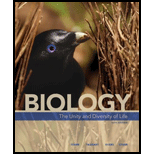Chapter 44, Problem 4SQ### Biology: The Unity and Diversity o...

15th Edition
Cecie Starr + 3 others
ISBN: 9781337408332

#### Solutions

Chapter
Section### Biology: The Unity and Diversity o...

15th Edition
Cecie Starr + 3 others
ISBN: 9781337408332
Textbook Problem
3 views

# A population of worms is growing exponentially in a compost heap. Thirty days ago there were 300 worms and now there are 600. How many worms will there be 30 days from now, assuming conditions remain constant and resources are unlimited? a. 1,200 b. 1,600 c. 3,200 d. 6,400

Summary Introduction

Concept introduction: The rise in number of individuals in a population is called population growth. Exponential growth is a pattern of data that increases greater with the increase in time at a consistent rate. The population growth rate becomes more rapid in proportion to the growing total size of population. If the population grows abruptly, it attains the peak value forming a J-shaped curve.

Explanation

Reasons for correct statements:

With the exponential growth, the population size increases at a consistent rate. In each interval, the number of individual added to the current population size is described using the following equation:

G=r*N

Where G is exponential growth.

r is the rate of growth with respect to time.

N is the number of individuals.

Here, the number of individuals is 600.

Thirty days before the number present is 300 and now, it is 600. Therefore, it is twice the original population. Therefore, the rate of change of population is 2.

G=2*600G=1,200

Therefore, The number of worms present there in 30 days from then with assuming conditions remain constant and resources are unlimited are 1,200

### Still sussing out bartleby?

Check out a sample textbook solution.

See a sample solution

#### The Solution to Your Study Problems

Bartleby provides explanations to thousands of textbook problems written by our experts, many with advanced degrees!

Get Started